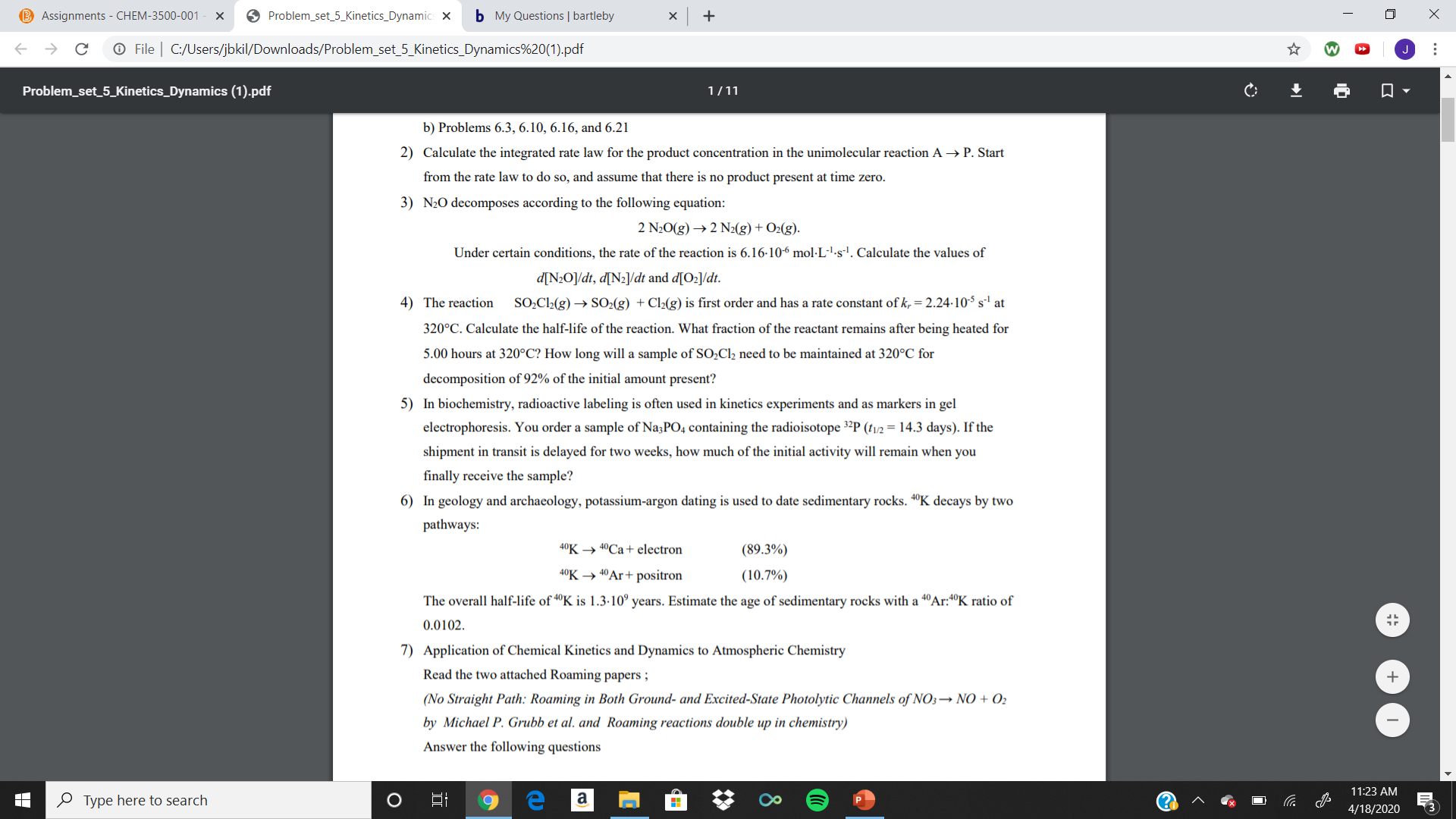# B Assignments - CHEM-3500-001 O Problem_set_5_Kinetics_Dynamic: X b My Questions | bartleby O File | C:/Users/jbkil/Downloads/Problem_set_5_Kinetics_Dynamics%20(1).pdf Problem_set_5_Kinetics_Dynamics (1).pdf 1/11 b) Problems 6.3, 6.10, 6.16, and 6.21 2) Calculate the integrated rate law for the product concentration in the unimolecular reaction A → P. Start from the rate law to do so, and assume that there is no product present at time zero. 3) N20 decomposes according to the following equation: 2 N20(g) → 2 N2(g) + O2(g). Under certain conditions, the rate of the reaction is 6.16-106 mol·L-'-s'. Calculate the values of d[N2O]/dt, d[Nz]/dt and d[O2]/dt. 4) The reaction SO,Cl2(g) → SO2(g) + Cl2(g) is first order and has a rate constant of k, = 2.24-10S s' at 320°C. Calculate the half-life of the reaction. What fraction of the reactant remains after being heated for 5.00 hours at 320°C? How long will a sample of SO;Cl2 need to be maintained at 320°C for decomposition of 92% of the initial amount present? 5) In biochemistry, radioactive labeling is often used in kinetics experiments and as markers in gel electrophoresis. You order a sample of Na;PO4 containing the radioisotope 3²P (tv/2 = 14.3 days). If the shipment in transit is delayed for two weeks, how much of the initial activity will remain when you finally receive the sample? 6) In geology and archaeology, potassium-argon dating is used to date sedimentary rocks. 4°K decays by two pathways: 40K → 40CA+ electron (89.3%) 40K → 40Ar+ positron (10.7%) The overall half-life of "K is 1.3-10° years. Estimate the age of sedimentary rocks with a 4°Ar:4°K ratio of 0.0102. 7) Application of Chemical Kinetics and Dynamics to Atmospheric Chemistry Read the two attached Roaming papers ; (No Straight Path: Roaming in Both Ground- and Excited-State Photolytic Channels of NO3→ NO + O2 by Michael P. Grubb et al. and Roaming reactions double up in chemistry) Answer the following questions 11:23 AM O Type here to search (? 4/18/2020 ...

Question

I need help on my study problems. I was wondering if you could help with 4. Thanks!help_outlineImage TranscriptioncloseB Assignments - CHEM-3500-001 O Problem_set_5_Kinetics_Dynamic: X b My Questions | bartleby O File | C:/Users/jbkil/Downloads/Problem_set_5_Kinetics_Dynamics%20(1).pdf Problem_set_5_Kinetics_Dynamics (1).pdf 1/11 b) Problems 6.3, 6.10, 6.16, and 6.21 2) Calculate the integrated rate law for the product concentration in the unimolecular reaction A → P. Start from the rate law to do so, and assume that there is no product present at time zero. 3) N20 decomposes according to the following equation: 2 N20(g) → 2 N2(g) + O2(g). Under certain conditions, the rate of the reaction is 6.16-106 mol·L-'-s'. Calculate the values of d[N2O]/dt, d[Nz]/dt and d[O2]/dt. 4) The reaction SO,Cl2(g) → SO2(g) + Cl2(g) is first order and has a rate constant of k, = 2.24-10S s' at 320°C. Calculate the half-life of the reaction. What fraction of the reactant remains after being heated for 5.00 hours at 320°C? How long will a sample of SO;Cl2 need to be maintained at 320°C for decomposition of 92% of the initial amount present? 5) In biochemistry, radioactive labeling is often used in kinetics experiments and as markers in gel electrophoresis. You order a sample of Na;PO4 containing the radioisotope 3²P (tv/2 = 14.3 days). If the shipment in transit is delayed for two weeks, how much of the initial activity will remain when you finally receive the sample? 6) In geology and archaeology, potassium-argon dating is used to date sedimentary rocks. 4°K decays by two pathways: 40K → 40CA+ electron (89.3%) 40K → 40Ar+ positron (10.7%) The overall half-life of "K is 1.3-10° years. Estimate the age of sedimentary rocks with a 4°Ar:4°K ratio of 0.0102. 7) Application of Chemical Kinetics and Dynamics to Atmospheric Chemistry Read the two attached Roaming papers ; (No Straight Path: Roaming in Both Ground- and Excited-State Photolytic Channels of NO3→ NO + O2 by Michael P. Grubb et al. and Roaming reactions double up in chemistry) Answer the following questions 11:23 AM O Type here to search (? 4/18/2020 ... fullscreen

### Want to see this answer and more?

Experts are waiting 24/7 to provide step-by-step solutions in as fast as 30 minutes!*

*Response times may vary by subject and question complexity. Median response time is 34 minutes for paid subscribers and may be longer for promotional offers.
Tagged in
Science
Chemistry

### Physical Chemistry